# How to Find the Equation of a Trend Line

An error occurred trying to load this video.

Try refreshing the page, or contact customer support.

Coming up next: What is a Variable in Algebra?

### You're on a roll. Keep up the good work!

Replay
Your next lesson will play in 10 seconds
• 0:00 Finding the Equation:…
• 4:33 Example
• 6:01 Lesson Summary

Want to watch this again later?

Timeline
Autoplay
Autoplay
Speed

#### Recommended Lessons and Courses for You

Lesson Transcript
Instructor: Yuanxin (Amy) Yang Alcocer

Amy has a master's degree in secondary education and has taught math at a public charter high school.

What is a trend line and how is it useful? That's what you'll learn, as well as the formulas you need to use and the numbers you need to find for the equation of a trend line, in this lesson. You'll also see what steps you need to take to manipulate the formulas to your desired equation.

## Finding the Equation: The Steps

In this lesson, we'll talk about finding the equation of a trend line. In the real world, your data will usually be scattered like in this graph instead of lining up neatly in a straight line.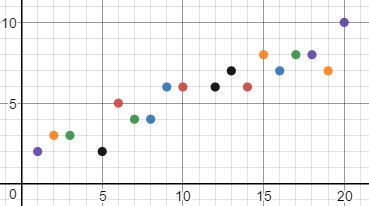In some real world cases, though, your data will look like it follows a line. If it does, then you'll be able to approximate your data with a line and a linear equation. This line that you approximate your data with is called the trend line. If this line is a straight line, then you'll be able to find an equation for this line.

In this lesson, we'll talk about finding an equation for trend lines that are straight lines. For these types of trend lines, you'll be able to find an equation in the slope-intercept form where m is your slope and b is your y-intercept. Remember, your slope is how steep your line is. A flat, horizontal line has a slope of 0. A diagonal line on the graph has a slope of 1. Steeper lines have larger slopes. Your y-intercept is where your line crosses the y-axis.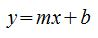To find your equation of a trend line, follow these steps.

#### Step 1: Draw your trend line.

You begin by drawing your trend line. You want your trend line to follow your data. You want to have roughly half your data above the line and the other half below the line, like this: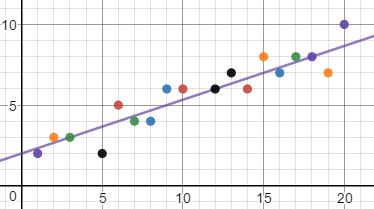#### Step 2: Locate two points on the line.

Your next step is to locate two points on the trend line. Look carefully at your trend line and look for two easy to figure out points on the line. Ideally, these are points where the trend line crosses a clearly identifiable location.

For the trend line that we just drew, we can see these two easily identifiable points.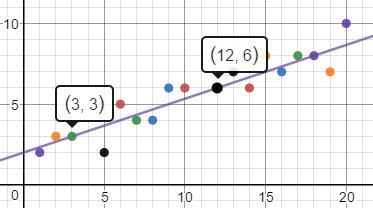We can easily identify these two points as (3, 3) and (12, 6).

#### Step 3: Plug these two points into the formula for slope.

The formula for slope is this one: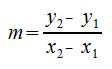We can label our first point as (x1,y1), and our second point as (x2,y2). So our x1 is 3, our y1 is 3, our x2 is 12, and our y2 is 6. Plugging these values into the equation for slope and evaluating, we get this: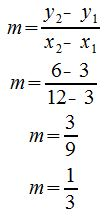So our slope is 1/3.

To unlock this lesson you must be a Study.com Member.

### Register to view this lesson

Are you a student or a teacher?

### Unlock Your Education

#### See for yourself why 30 million people use Study.com

##### Become a Study.com member and start learning now.
Back
What teachers are saying about Study.com

### Earning College Credit

Did you know… We have over 160 college courses that prepare you to earn credit by exam that is accepted by over 1,500 colleges and universities. You can test out of the first two years of college and save thousands off your degree. Anyone can earn credit-by-exam regardless of age or education level.